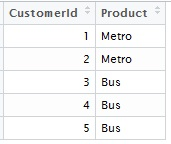## Pages

### Full, Innner, Left and Right Joins in R

In the previous example, the matching keys were same across the datasets. What if the situation is not that straight forward.

Let's face the first complication.

# Let's first clear the workspace, else R interface looks too clutteredData_1
rm(list = ls())

#Let' now create two datasets

Data_1 = data.frame(CustomerId = c(1:5), Product = c(rep("Metro", 2), rep("Bus", 3)))Data_2

Data_2 = data.frame(CustomerId = c(2, 4, 6), City = c(rep("Delhi", 2), rep("Mumbai", 1)))

### We shall now try 4 variants of the tables joins

# First full join : which is A union B i.e. All the components be it in A or B

Data_full =  merge(x = Data_1 , y = Data_2, by = "CustomerId", all = TRUE)

# Inner join : Which is nothing but A intersection B i.e. components common in A and B

Data_inner =  merge(x = Data_1 , y = Data_2, by = "CustomerId")

# Left join : Keeping A fixed i.e. All the components of A irrespective of that of B

Data_left =  merge(x = Data_1 , y = Data_2, by = "CustomerId", all.x = TRUE)

# Left join : Keeping B fixed i.e. All the components of B irrespective of that of A

Data_right =  merge(x = Data_1 , y = Data_2, by = "CustomerId", all.y = TRUE)